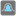• # I need a tip to solve that mission

## Question related to missionProbably Dice

Hi everyone,

I'm just testing and programming for hours and I don't find a solution, which is both efficient and precise :/

At the moment I've got two approaches:

-> SOLUTION BY BRUTE-FORCE:

```result = 0
rolls = int(1e8)
for i in range(rolls):
roll = [randint(1,6) for x in range(dice_number)]
if sum(roll) == target:
result += 1
return round( result/rolls, 4)
```

-> SOLUTION BY CALCULATING THE PRECISE RESULT:

```dice = [x for x in range(1,sides+1)]
candidates = [p for p in product(dice, repeat=dice_number) if sum(p) == target]
return round(len(candidates) / (sides**dice_number), 4)
```

Pls, could you give me a hint to solve that?